# CREST Mathematics Olympiad Class Prep/KG Sample Papers

## Syllabus:

Section1: Basic Concepts on Comparison (Big-Small, Front-Back, Full-Empty, Heavy-Light, In-Out, More-Less, Right-Left, Same-Different, Tall-Short, Thick-Thin, Top-Bottom)
Numbers (Number names (1-50), Before, After, Between Numbers (1-20), Skip Counting (2 and 5))
Playing Numbers (1-digit addition and subtraction, Putting Signs like \'>\', \'<\' and \'=\')
Time (Days of a week, Months in a year)
Shapes (Square, Rectangle, Triangle, Circle, Oval, Sphere, Cone, Cube, Cylinder)

Achievers Section: Higher Order Thinking Questions - Syllabus as per Section 1

 Q.1 What is the shape of the given image?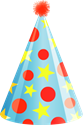Q.2 Find the missing number: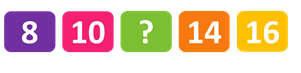Q.3 Which number is given in the image?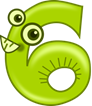Q.4 Identify the bird which is facing towards right:
 Q.5 Which clock indicates 6:00?
 Q.6 Identify the circle:
 Q.7 Which one is the back image of the given image: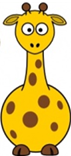Q.8 Find the difference: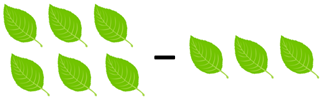Q.9 Complete the series: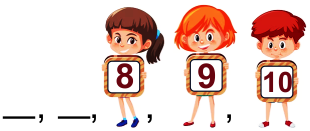Q.10 Find the sum of next two numbers in the pattern: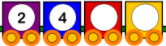Sample PDF of CREST Mathematics Olympiad for Class Prep/KG: